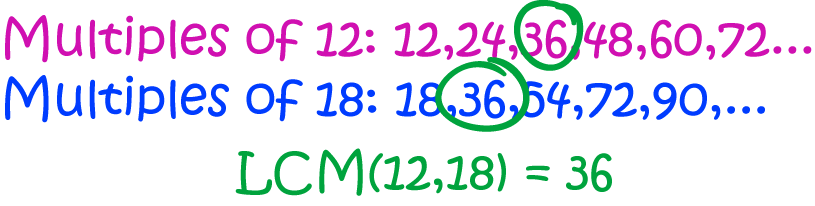Definition of Least Common MultipleThe lowest common multiple of two integers is the smallest positive integer that both numbers are factors of. In other words, it is the smallest positive integer that is a multiple of both numbers. We can similarly find the lowest common multiple of more than two numbers.

For example, to find the lowest common multiple of $12$ and $18$, we start by writing down lists of numbers, in increasing order, that are multiples of each number. The lowest common multiple is the smallest number that occurs in both lists. So, the lowest common multiple of $12$ and $18$ is $36$.

Note that $72$ is also a common multiple of $12$ and $18$, but it is not the lowest common multiple, because $36$ is a common multiple that is smaller.

Note: Before we start writing down multiples of $12$ and $18$, we know that these numbers have one common multiple: $12 \times 18 = 216$, so we know our lists of common multiples will eventually stop.

Another term for "lowest common multiple" is "least common multiple".

Description

The aim of this dictionary is to provide definitions to common mathematical terms. Students learn a new math skill every week at school, sometimes just before they start a new skill, if they want to look at what a specific term means, this is where this dictionary will become handy and a go-to guide for a student

Audience

Year 1 to Year 12 students

Learning Objectives

Learn common math terms starting with letter L

Author: Subject Coach